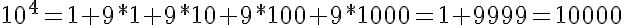I just finished a great book on Fermat's Last Theorem.
Fermat's Enigma

It was very well written and I enjoyed it because it explored each great mathematician from Pythagoras up through Andrew Wiles (who solved the problem). I would recommend the book to anyone interested in math, especially those who enjoy number theory.

For those of you who don't know much about number theory, it's a branch of mathematics dealing with the property of numbers. Just like how chemists examine oxygen, carbon, etc. to see how they interact with each other, number theorists examine how numbers interact. For example, there are certain numbers called perfect numbers. 6 is one of them. The factors of 6 (1,2,3) add up to 6. Numbers have properties such as being prime, perfect squares, odd, even. These don't seem to be assigned at random. There is a pattern to many of these, and number theorists try to discover these patterns. The book is about one particular relation, and how it took over 300 years to prove.

In reading it, I came up with a few theorems and proofs that I found interesting. While not technically difficult, they caught my attention.

In reading it, I came up with a few theorems and proofs that I found interesting. While not technically difficult, they caught my attention.This is most clearly demonstrated with:But is in fact true for all values of a and x. The proof by induction is quite simple.

The other problem I've been exploring has less to do with equations and is more of a simple puzzle. It's called "The Broken Weight Problem". The idea is that using a standard balance, what is the minimum number of weights needed to weigh anything of whole values between 1 and 40.

The initial thought is that you need 6 weights, values 1,2,4,8,16, and 32. This is based on the assumption that you can only add weights to one side of the balance.

The actual solution is that you need 4 weights, values 1, 3, 9, and 27. This only works if you add these weights to both sides to get a balanced measurement. For example, to measure an orange of weight 2 you put the orange and a 1 lb weight on one side and balance it with a 3 lb weight.

The first solution is the powers of 2, the second is the powers of 3. So I started thinking about a scenario in which the solution would be the powers of 4.Consider a balance like the one above.   The object to be weighed is placed in B.  The weights can be placed in A, B, or C.  The weights in C would have a factor of 2 applied due to leverage.  In this scenario, the only weights needed would be 1, 4, 16, 64, etc.  (The powers of 4).   So to get a number like 35, one could put 16 and in C and  4 in A, totaling 36 on the left.  Put the object being weighed and the 1 weight in B, balancing the scale.In this case, D would be a counter balance.  The weights in D would also have a factor of 2.  In this scenario, the weights needed would be 1, 5, 25, 125, etc. (The powers of 5).

I don't have a proof for this, but I suspect this pattern continues as you add arms to the balance.  The next set would have a spot for weights on the left side that would be a factor of 3 and would result in the powers of 6 being the minimum set of weights.  The following set would add an arm on the right side with a factor of three.  This would result in the powers of 7 being the minimum set of weights.

Why is this important at all?  It's not really.  But I found it to be an interesting pattern.  There are probably some applications in number theory dealing with various bases, and I may try to write it up as some equations someday and try to prove it.  But this is enough for now.